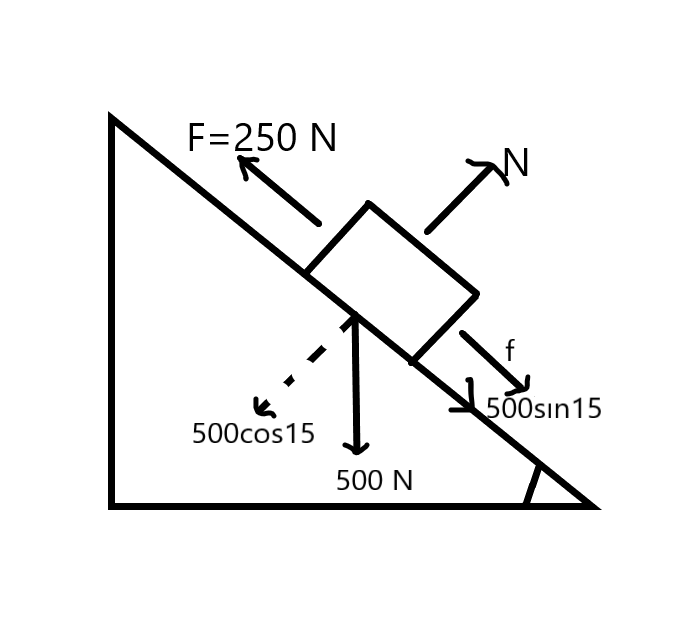# A force of 250 N pulls a body of weight 500 N up an inclined plane, the force being applied parallel to the plane. If the inclination of the plane to the horizontal is 15°, find the coefficient of friction.

Question-AnswerCategory: Engineering MechanicsA force of 250 N pulls a body of weight 500 N up an inclined plane, the force being applied parallel to the plane. If the inclination of the plane to the horizontal is 15°, find the coefficient of friction.

A force of 250 N pulls a body of weight 500 N up an inclined plane, the force being applied parallel to the plane. If the inclination of the plane to the horizontal is 15°, find the coefficient of friction.

Step: 1

Force, F = 250 N pulling the body of weight
W = mg = 500 N up an inclined plane parallel to the plane which makes an angle 15o with the horizontal.Step: 2

From the figure we can make equations,
N = 500cos15
250 = f+500sin15    (f=uN is the frictional force)
u 500 cos15 = 250500sin15
u=120.6/500cos15  = 0.966